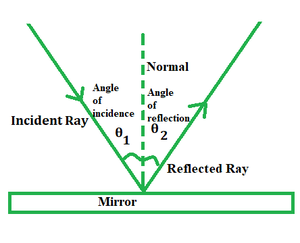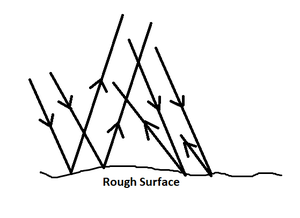# Reflection and Ray Model of Light Formula

Reflection of light is a very important concept in physics. After all, this phenomenon is what allows us to see the world around us. In this article, the reflection of light is discussed.

Law of Reflection

A ray of light(incident) falling on a polished surface is shown to bounce back after striking the said surface at the same angle it struck the surface. The ray after bouncing back is termed the reflected ray and the angle at which it does so is called the angle of reflection.

The normal to the perpendicular is what separates the incidence and reflection angles into equal magnitudes.Examples

• Assume you light a torch in a dark room and make the light fall at an angle against a mirror placed on the ground. You will notice a ray of light bouncing towards the direction opposite to the incident ray.
• Lighting a candle in a dark room lets you see things around that you were not able to see earlier. Why so? This is because the light emitted from the candle falls onto the objects and then bounces back to your eyes to let you see them.

Formula

θ1 = θ2

where θ1 and θ2 represent the angles of incidence and reflection respectively.

### Sample Problems

Problem 1. A ray of light strikes a surface at an angle of 70° to the normal. At what angle would it be reflected?

Solution:

Since, θ1 = θ2 as per the law of reflection.

Here, θ1 = 70°

⇒ θ2 = 70°

Problem 2. How would you explain the reflection of light to a layman?

Solution:

In simple words, reflection of light can be explained as the phenomenon whereby upon hitting a surface, the ray does not enter a whole new medium, instead it bounces off at the angle it hit the said surface.Problem 3. What do you mean by diffuse reflection?

Solution:

Diffuse reflection can be described as an overlapping of all reflected rays. When a ray of light strikes a rough or irregular surface, the rays do get reflected at each angle of inflection with the normal, but their visual effect gets ‘cancelled’ because of overlapping.Problem 4. A ray of light strikes a surface at an angle of 30° to the normal. At what angle would it be reflected?

Solution:

Since, θ1 = θ2 as per the law of reflection.

Here, θ1 = 30°

⇒ θ2 = 30°

Problem 5. A ray of light strikes a surface at an angle of 40° to the normal. At what angle would it be reflected?

Solution:

Since, θ1 = θ2 as per the law of reflection.

Here, θ1 = 40°

⇒ θ2 = 40°

Whether you're preparing for your first job interview or aiming to upskill in this ever-evolving tech landscape, GeeksforGeeks Courses are your key to success. We provide top-quality content at affordable prices, all geared towards accelerating your growth in a time-bound manner. Join the millions we've already empowered, and we're here to do the same for you. Don't miss out - check it out now!

Previous
Next